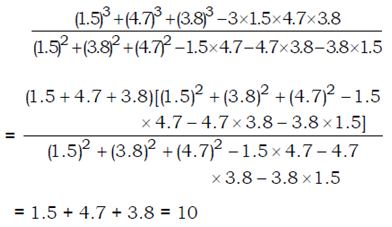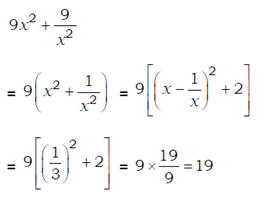# SSC CGL 2018 Practice Test Papers | Quantitative Aptitude (Day-22)

Dear Aspirants, Here we have given the Important SSC CGL Exam 2018 Practice Test Papers. Candidates those who are preparing for SSC CGL 2018 can practice these questions to get more confidence to Crack SSC CGL 2018 Examination.

[WpProQuiz 2992]

Click “Start Quiz” to attend these Questions and view Explanation

1) Simplify:

112÷2×13÷5 0.6 15 + 1=?

a) 130

b) 120

c) 131

d) 129

2) A contractor deploys 36 men on a work and in 16 days they complete 2/3 rd of the work.To finish the remaining work he deploys 12 men extra. Now the remaining work will be completed in _________

a) 10 days

b) 5 days

c) 10 days

d) 6 days

3) If 1/a, 1/b and 1/c are in arithmetic progression,then which one of the following statement is true?

a)(a/c) = (a–b)/(b–c)

b)b^2 = ac

c)(a/b) = (c/a)

d)(c/a) = (a–b)/(b – c)

4) Two numbers are in the ratio 3 : 5. If 8 is added to each number, they will be in the ratio 5 : 7. What is the product of the two numbers?

a) 240

b) 200

c) 300

d) 360

5)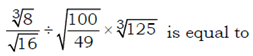a) 1 3/4

b) 7

c) 9

d) 10

6) An article was purchase at a cost of 3/4 of certain price. It is marked 40% above the cost price and it was sold at 20% discount. Find the profit Percentage?

a) 42

b) 49 1/3

c) 47 1/2

d) 50

7) The average of A and B is 40, average of B and C is 14 and average of C and A it is 19. Then what is the value of A?

a) 10

b) 13

c) 25

d) 20

8) The speed of a motorboat in still water is 22 km/hr. If the speed of the stream is 4.5 km/hr, then the ratio of the time taken in going a distance of 53 km along the stream and 70 km distance against the stream is_____

a) 1:2

b) 2:3

c) 3:1

d) 1:3

9) The value of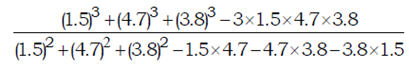a) 11

b) 30

c) 20

d) 10

10)a) 24

b) 25

c) 19

d) 20

112÷2×13÷5 − 0.6 −15+1=131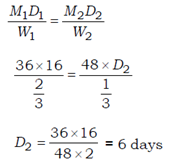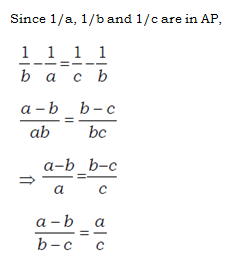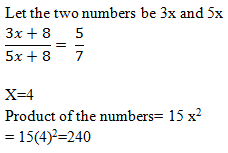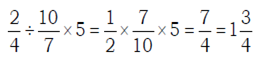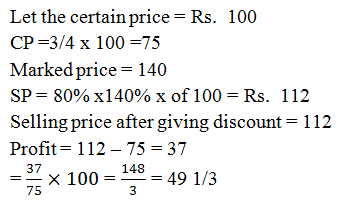A+B = 40×2 = 80 —– (I)

B+C = 14×2 = 28 —— (II)

C+A = 19×2 =38 ——- (III)

On solving I, II and III

Then A=25

Speed downstream = (22 + 4.5) km/hr = 26.5 km/hr

Time taken in going a distance of 53 km along the stream =53/26.5 = 2 hours

Speed upstream = (22 – 4.5)

= 17.5 km/hr

Time taken in going 70 km against the stream = 70/17.5 = 4 hours

Required ratio =2/4

= 1 : 2